# (d) A particle of mass m is moving at a speed u and hits an identical particle that is siting at ...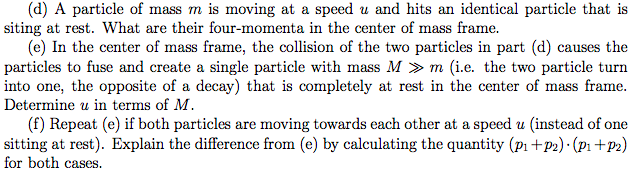(d) A particle of mass m is moving at a speed u and hits an identical particle that is siting at rest. What are their four-momenta in the center of mass frame (e) In the center of mass frame, the collision of the two particles in part (d) causes the particles to fuse and create a single particle with mass M »m (i.e. the two particle turn into one, the opposite of a decay) that is completely at rest in the center of mass frame. Determine u in terms of M. (f) Repeat (e) if both particles are moving towards each other at a speed u (instead of one sitting at rest). Explain the difference from (e) by calculating the quantity (Pi +P2) (pi+P2) for both cases.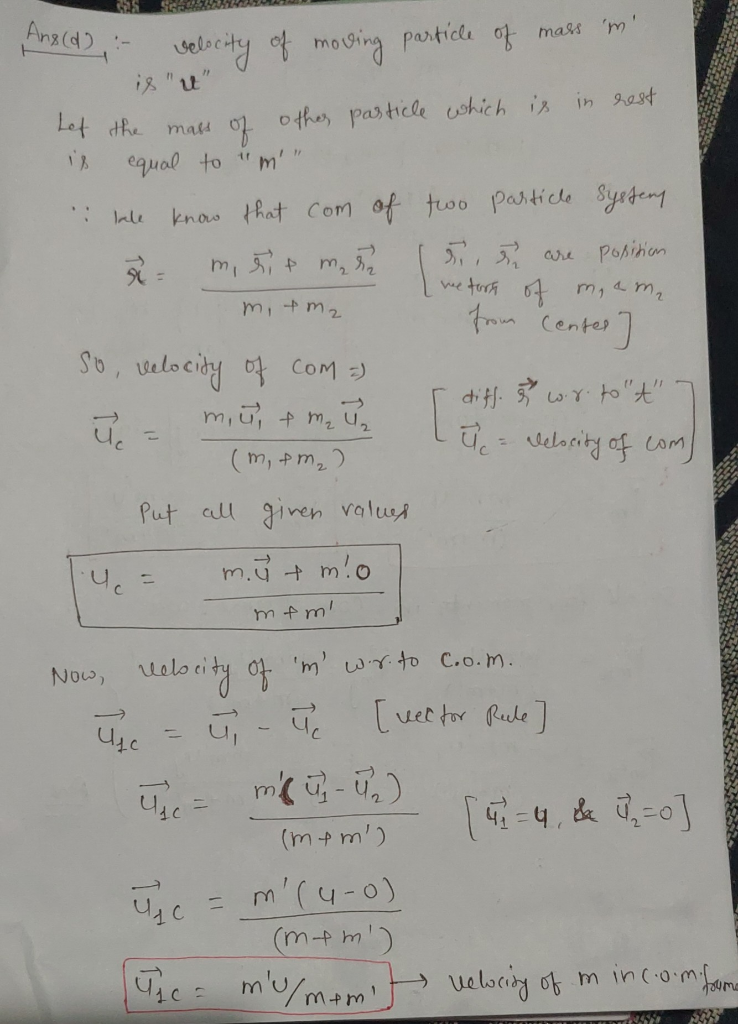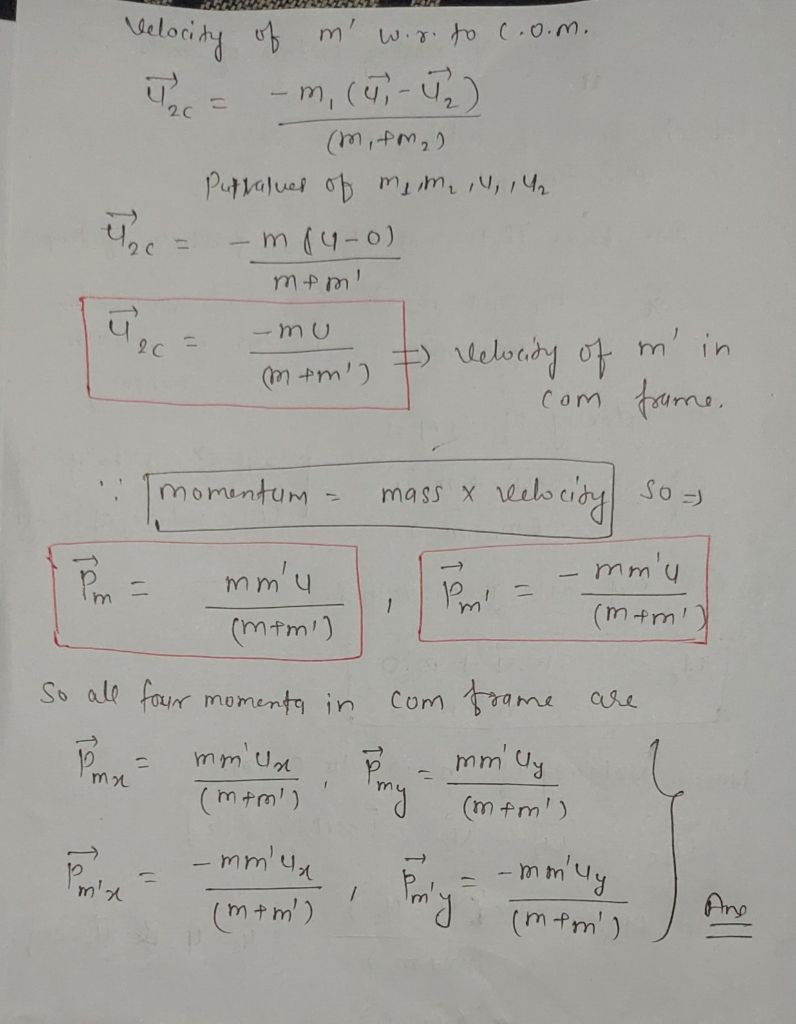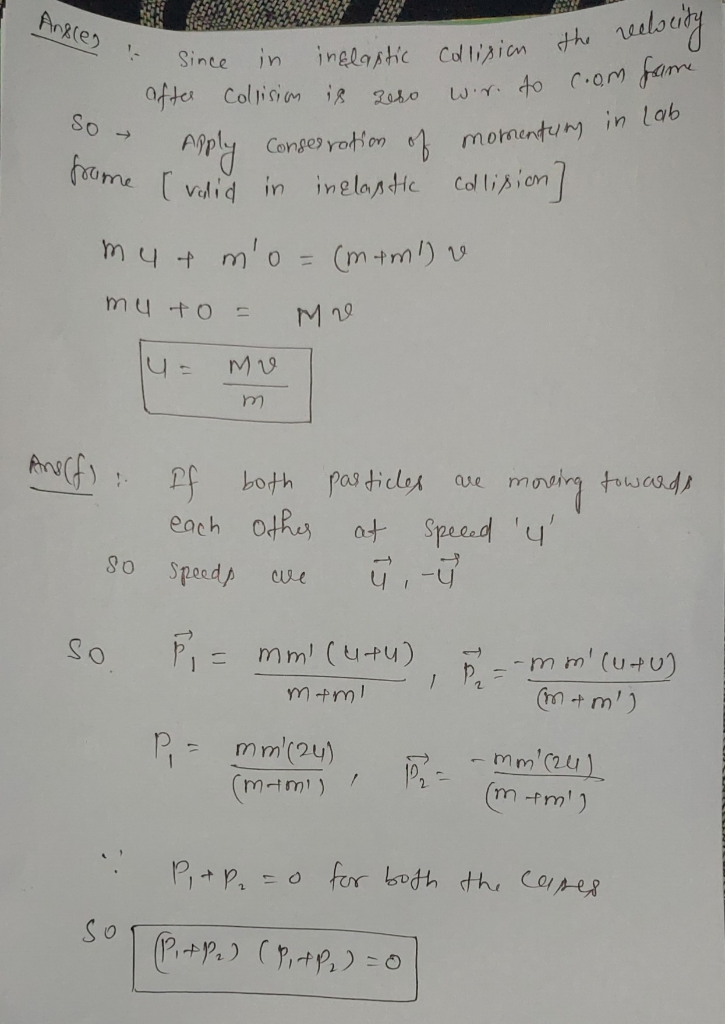##### Add Answer of: (d) A particle of mass m is moving at a speed u and hits an identical particle that is siting at ...
Similar Homework Help Questions
• ### 2) A particle of mass m at rest in the Home frame decays into two particles of mass that move apa...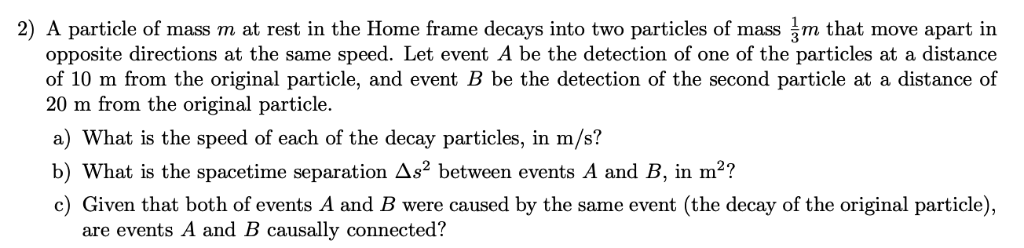2) A particle of mass m at rest in the Home frame decays into two particles of mass that move apart in opposite directions at the same speed. Let event A be the detection of one of the particles at a distance of 10 m from the original particle, and event B be the detection of the second particle at a distance of 20 m from the original particle. a) What is the speed of each of the decay particles,...

• ### 3. A particle of rest mass m moving in the a direction at a speed of...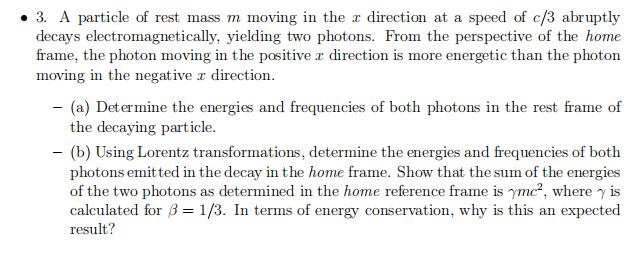3. A particle of rest mass m moving in the a direction at a speed of c/3 abruptly decays electromagnetically, yielding two photons. From the perspective of the home frame, the photon moving in the positive r direction is more energetic than the photon moving in the negative r direction - (a) Determine the energies and frequencies of both photons in the rest frame of the decaying particle. -(b) Using Lorentz transformations, determine the energies and frequencies of both photons...

• ### Modern Physics Particle A. of mass 0.511MeVle',mOves at 0.00 and collides with particle B of mass...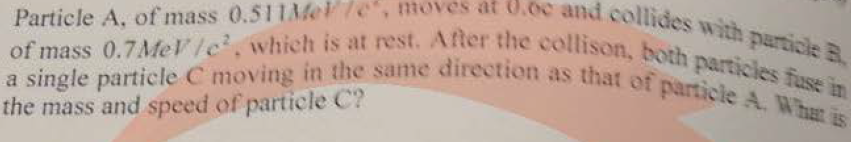Modern Physics Particle A. of mass 0.511MeVle',mOves at 0.00 and collides with particle B of mass 0.7MeV/e which is at rest. After the collison, both particles fuse in a single particle C moving in the same direction as that of particle A. Wh is the mass and speed of particle C?

• ### Bonus problem A particle of m, is moving horizontally with vi, and collides with a particle...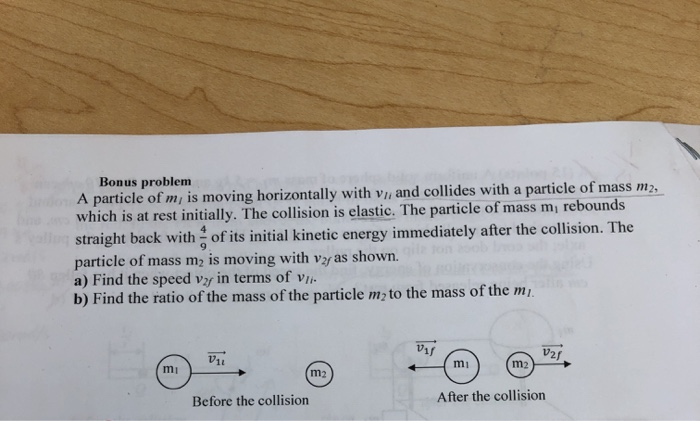Bonus problem A particle of m, is moving horizontally with vi, and collides with a particle of mass m2, which is at rest initially. The collision is elastic. The particle of mass mrebounds straight back with of its initial kinetic energy immediately after the collision. The particle of mass me is moving with vas shown. a) Find the speed vz in terms of viin b) Find the ratio of the mass of the particle m to the mass of the...

• ### Problem (3) A particle of mass M is at rest in the laboratory when it decays into three identical...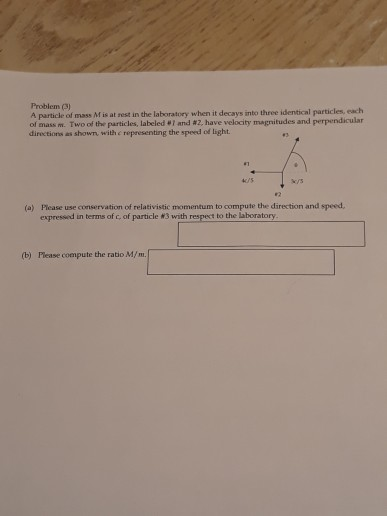Problem (3) A particle of mass M is at rest in the laboratory when it decays into three identical particles, each of mass m. Two of the particles, labeled #1 and #2, have velocity magnitudes and perpendicular directions as shown, with e representing the speed of light. Please use conservation of relativistic momentum to compute the direction and speed, expressed in terms of c, of particle #3 with respect to the laboratory (a) (b) Please compute the ratio M/m. Problem...

• ### On a frictionless surface. a block of mass M moving at speed v collides elastically with...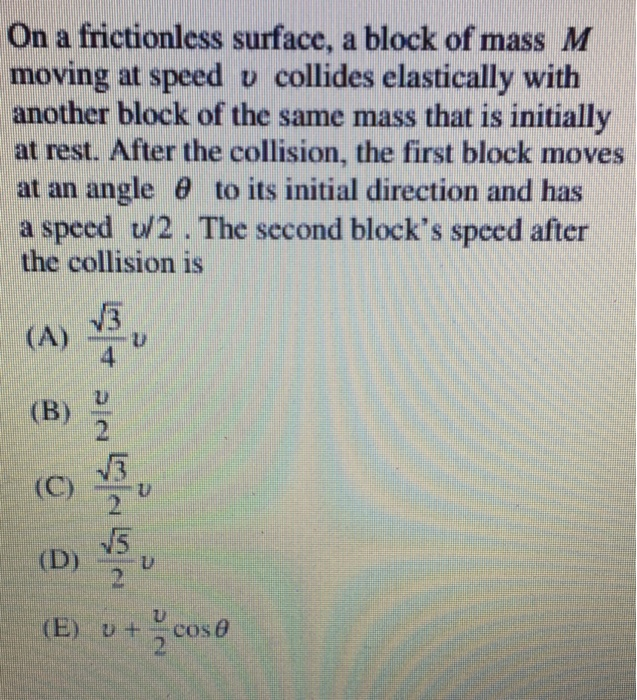On a frictionless surface. a block of mass M moving at speed v collides elastically with another block of the same mass that is initially at rest. After the collision, the first block moves at an angle θ to its initial direction and has a speed U/2. The second block's speed after the collision is 3 4 (B) 2 2 U) (E) ut cose

• ### A ball of mass 0.180 kg moving with speed 1.87 m/s hits a wall and bounces...

A ball of mass 0.180 kg moving with speed 1.87 m/s hits a wall and bounces back with speed 1.00 m/s in the opposite direction. What is the change in the ball’s kinetic energy?

• ### PHYS10121 a) A particle of rest mass m is travelling so that its total energy is...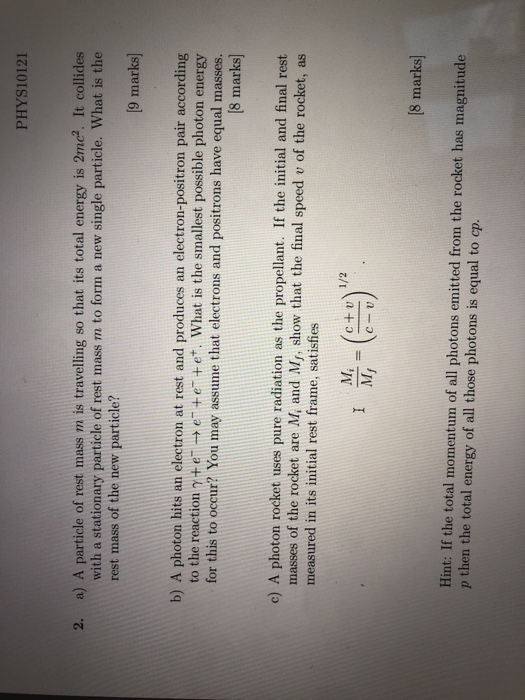PHYS10121 a) A particle of rest mass m is travelling so that its total energy is 2mc. It collides with a stationary particle of rest mass m to form a new single particle. What is the 2. rest mass of the new particle? 9 marks] b) A photon hits an electron at rest and produces an electron-positron pair according to the reaction γ+ e- e" + e-+e+, what is the smallest possible photon energy for this to occur? You may...

• ### A bullet of mass 0.01 kg is moving horizontally with a speed of 100 m/s when it hits a block of mass 2 kg that is at rest on a horizontal surface with a coefficient of friction of 0.4

A bullet of mass 0.01 kg is moving horizontally with a speed of 100 m/s when it hits a block of mass 2 kg that is at rest on a horizontal surface with a coefficient of friction of 0.4. After the collision the bullet becomes embedded in the block.

• ### QUESTION 7 A bullet of mass 40.0 g moving with a speed of 249 m/s strikes...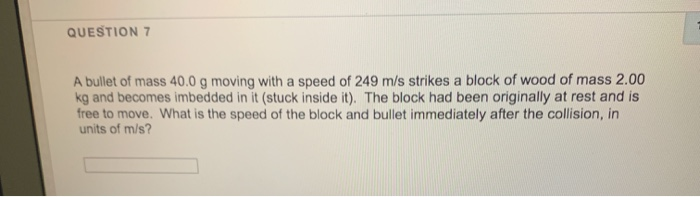QUESTION 7 A bullet of mass 40.0 g moving with a speed of 249 m/s strikes a block of wood of mass 2.00 kg and becomes imbedded in it (stuck inside it). The block had been originally at rest and is free to move. What is the speed of the block and bullet immediately after the collision, in units of m/s? QUESTION 10 If an object is initially at rest and then 'explodes' into two separate pieces, what do we...

Need Online Homework Help?